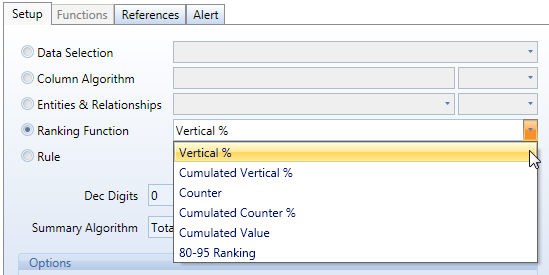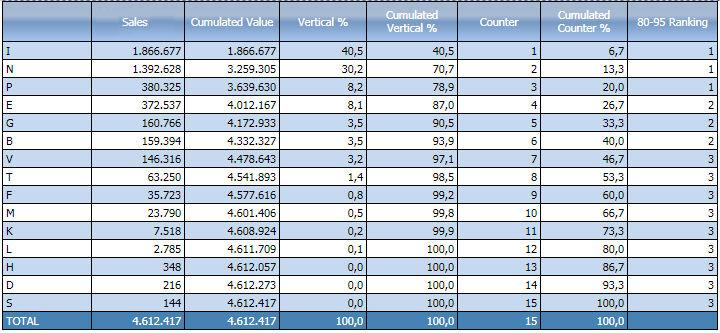﻿ Ranking function

# Ranking function

Ranking functions are pre-defined functions which allow to quickly create ranking analysis.

## List of available ranking functions

Vertical %. Returns the percentage value of the current row on the column total.

Cumulated Vertical %. Cumulates the Vertical% value from top-down. This function should be applied on sorted reports, go to the Filters tab to apply ascending or descending sorting on columns.

Counter. Row counter, counts rows sequentially.

Cumulated Counter %. Returns the row number divided by the total number of rows as a percentage.

Cumulated Value. Cumulates a values starting from the top row, i.e. each row is calculated by summing the value of the above row plus the current row value.

80-95 Ranking. Creates three categories based on the cumulated vertical % value: the value is less than 80%, it returns 1, if it is between 80% and 95% it returns 2, if it is over 95%, it returns 3. This function should be applied on sorted reports, go to the Filters tab to apply ascending or descending sorting on columns.

Notes

• Ranking functions are applied on the block prior to where they are set therefore you cannot set a ranking function in the first block of a Layout (block "a").

• To use a ranking function, the layout must have an entity set by row.## Example of an analysis using Ranking functionsobtained with the following Layout:

block (a) : Sales cube: shows the sales amount per product, rows are sorted in descending order.

block (b) : Cumulated Value : accumulates row by row the sales values.

block (c) : Vertical % : returns the percentage of each row on the column total value.

block (d) : Cumulated Vertical % : returns the cumulated percentage of each row on the column total value.

block (e) : Counter  : counts the rows of the layout.

block (f) : Cumulated Counter % : returns the cumulated percentage of the row counter.

block (g) : 80-95 Ranking : returns 1 on the rows where the cumulated vertical % is lesser or equal to 80% (this is the top tier), returns 2 on the rows where the cumulated vertical % is between 80% and 95% (this is the second tier of items) and returns 3 for the remaining rows (last tier).